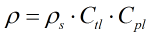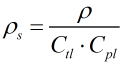# C294 – GPA TP-25/27 – NGL and LPG Density Referral Calculation

## Description

This calculates the density of an NGL or LPG at standard conditions or at process temperature and pressure using the guidelines set out in the below GPA and API standards.

GPA TP-25/API MPMS Chapter 11.2.4 – Temperature Correction for the Volume of Light Hydrocarbons – Tables 24E and 23E (1998)

GPA TP-27/API MPMS Chapter 11.2.4 – Temperature Correction for the Volume of NGL and LPG – Tables 23E, 24E, 53E, 54E, 59E and 60E (2007)

Measurement of Petroleum Measurement Standards Chapter 11.2.1 – Compressibility Factors for Hydrocarbons: 0-90° API Gravity Range (1984)

API MPMS Chapter 11.2.1M – Compressibility Factors for Hydrocarbons: 638 – 1074 Kilograms per Cubic Metre Range (1984)

Measurement of Petroleum Measurement Standards Chapter 11.2.2 – Compressibility Factors for Hydrocarbons: 0.350-0.637 Relative Density (60°F/60°F) and -50°F to 140°F Metering Temperature (First Edition October 1986)

API MPMS Chapter 11.2.2M – Compressibility Factors for Hydrocarbons: 350 – 637 Kilograms per Cubic Metre Density (15°C) and -46°C to 60°C Metering Temperature (1986)

API MPMS Chapter 11.1 – Temperature and Pressure Volume Correction Factors for Generalized Crude Oils, Refined Products and Lubricating Oils w/Addendum (2007)

GPA TP-15/API MPMS Chapter 11.2.5 – A Simplified Vapor Pressure Correlation for Commercial NGLs (2007)

Kelton calculation reference C294

KIMS calculation reference K229

## Options

### Standard

• GPA TP-25
• GPA TP-27

This option is used to select the standard from which the calculation is to be derived.

### Solve for

• Density
• Standard density

This option is used to select whether the calculation will solve for density at process or standard conditions.

### Precision

• Full precision
• Full rounding
• Rounding of inputs

This option is used to decide upon the rounding procedure to be employed. The full precision calculation doesn’t round any values. Full rounding rounds the inputs and outputs as described in the relevant standards. Rounding of inputs only rounds the input temperature and density according to the procedure set out in GPA TP-25 and 27. Note that the range of the density inputs at standard conditions vary from one standard temperature to another. Consult the relevant standards for details of the range of the input parameters of the calculation as the ranges associated with standards used in this procedure do not overlap at all temperatures and densities. Note that when rounding is used for certain input values the calculation does not converge, this issue is overcome by selecting full precision. An intermediate will be displayed to show whether the calculation has met convergence criteria.

### Vapour Pressure

• User entered
• Calculated using TP-15

This option is used to select vapour pressure is user ented or calculated according to the TP-15 standard. If user entered is selected a default value of 1 atmosphere (1.01325 bar) will be used unless a value is specified by the user.

### API Compressibility

• Metric
• US Customary

This option is used to select whether the pressure correction is to use the metric versions of API compressibility standards, MPMS Chapters 11.2.1M or 11.2.2M, or the standards in US customary units 11.2.1 and 11.2.2.

### Standard Temperature

• 15°C
• 20°C
• 60°F

If the TP-27 standard is selected then a choice of standard temperature is available. Note that TP-25 uses a standard temperature of 60°F.

## Calculation

### Temperature Correction

The temperature correction, Ctl, is calculated using the procedure as described in C293 – GPA TP-27:2007 – Temperature Correction for NGL and LPG. Note that the procedure used in TP-27 is the same as that of TP-25 but the temperature range has been adjusted and the procedure has been extended to be able to deal with densities at 15°C or 20°C standard temperature.

### Pressure Correction

The pressure correction used will depend on the standard selected and the value of the density at 15°C.

• If the TP-27 standard is selected and density at 15°C is greater than 637kg/m3 then API MPMS Chapter 11.1 is used to calculate the pressure correction.

The following table shows which standards are employed in each of the four scenarios.

 TP-25 TP-27 ρ15 > 637kg/m³ API Ch11.2.1/1M API Ch11.1 ρ15 ≤ 637kg/m³ API Ch11.2.2/2M API Ch11.2.2/2M

### Density

The density at temperature and pressure is calculated from the relationship:The process for calculating density at line conditions combines the relevant Ctl and Cpl calculations depending on the options set and the users input.

### Standard Density

The standard density is calculated using an iterative sequence using direct substitution. The standard density itself is calculated from the relationship:Note that there are a variety of reasons why this calculation will fail to calculate. The following is a non-exhaustive list of common reasons for such

• The critical temperature algorithms used by TP-25 and 27 differ to the one used by API MPMS Chapter 11.2.2M and have different failure criterion. The critical temperature criterion can be passed according to the TP-25/27 standards but fail due to the demands of the Chapter 11.2.2M procedure. If both criteria are not met the calculation will return not a number (NaN)
• The range of temperature permitted by each of the six standards used are all different from one another. The input temperature must be allowable by all of the in use standards.
• If the density is out of range specified in TP-25/27 the calculation will return not a number (NaN). There is also an intermediate that will return 0 if standard density is out of range.
• Due to the differing rounding procedures in some cases the calculation will not converge around the limits of the calculation at lower densities. The iterations converged intermediate will display 1 if convergence is achieved and 0 if it is not. If the calculation does not converge select full precision as this is not hindered by the differing rounding procedures.

### Vapour Pressure

If Vapour pressure is calculated it is done within the iterative process used above and is carried out according to the new method outlined in GPA TP-15. If calculated the vapour pressure is lower than atmospheric pressure then equilibrium pressure will be set at 1.01325 bar otherwise the vapour pressure will be used as the equilibrium pressure in the calculation of the Cpl.

Back